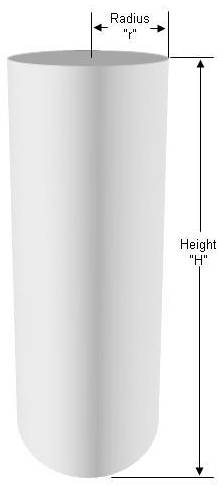Calculating The Amount Of Concrete Needed For A Column, Sonotube or Round Footing

Calculating the amount of concrete you require for a column, sono tube or round footing can be confusing.Notes:

• Pre-mix concrete comes in bags that are 60 lbs. and 80 lbs.
• A 60 lb. bag provides 0.45 cubic feet of cured concrete.
• A 80 lb. bag provides 0.6 cubic feet of cured concrete.
• If you have concrete delivered it is sold by the yard (which is a cubic yard = 27 cubic feet).

Example:

A column 8' high where the column has a radius of 5″ (10″ diameter tube)

3.14 x 5 x 5 x 96 ÷ 1728 = 4.4 cubic feet of concrete

4.4 ÷ 0.45 = 10 (9.8) 60 lb bags of concrete

4.4 ÷ 0.6 = 8 (7.3) 80 lb. bags of concrete

4.4 ÷ 27 = 0.16 cubic yards of concrete